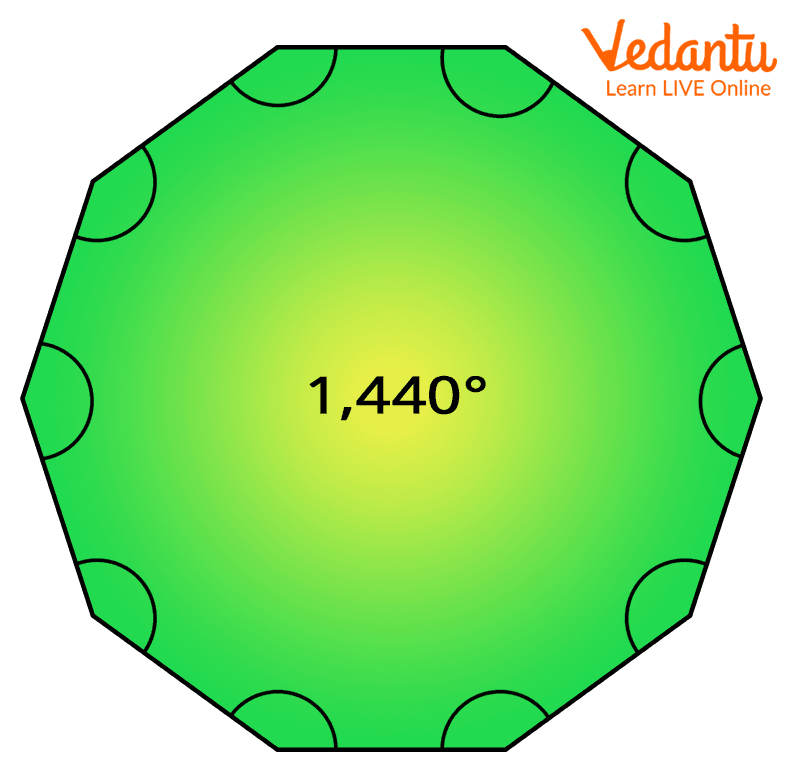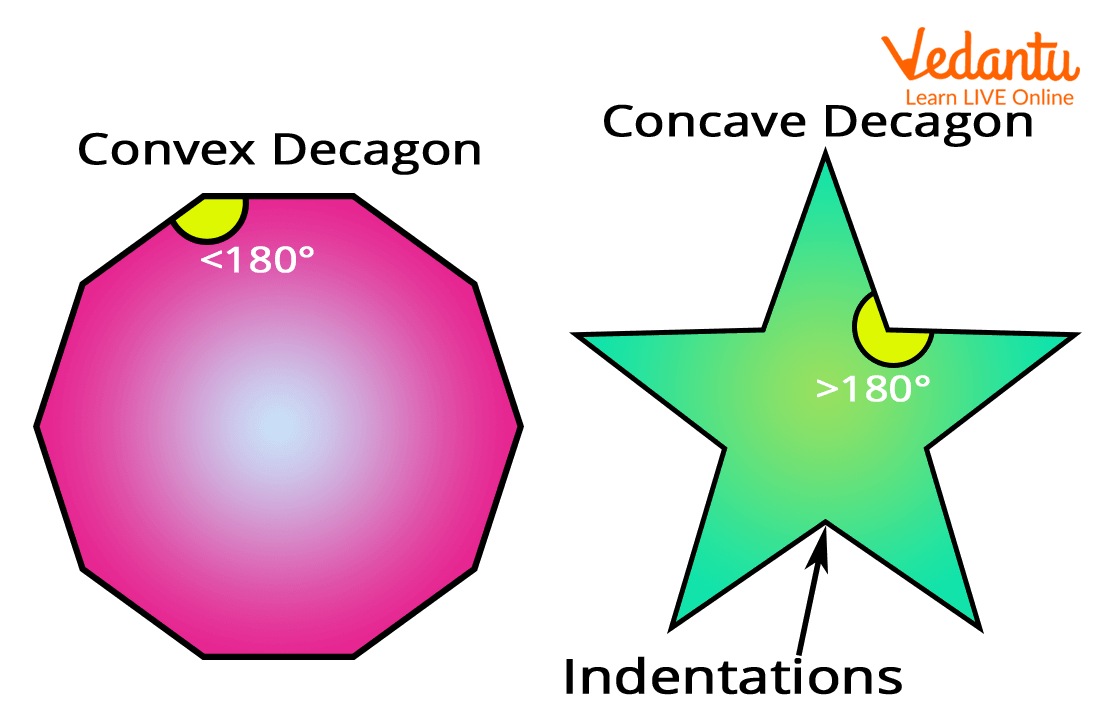Courses
Courses for Kids
Free study material
Free LIVE classes
More

# Sides of a DecagonLIVE
Join Vedantu’s FREE Mastercalss

## How Many Sides A Decagon Has?

Have you ever wondered what would a shape look like if it had ten sides? We, on our every day life, see different shapes, for example, a window has four sides, a triangle has three sides and a pentagon has five sides. Similarly, a shape having 10 sides is called a decagon. We also define decagon as the polygon having ten sides, ten interior angles, ten exterior angles, and ten vertices.

For example, the shape of a star has 10 sides, 10 vertices, which clearly indicates that the star is a decagon. Various types of decagons we study in mathematics. Here, on this page, we will focus on three types which are regular and irregular decagon, convex and concave decagon, and simple and complex decagon.

Now, let us start with the definition of a decagon and proceed with its formula for finding the number of sides and angles.

## What is a Decagon: Definition and Properties

From the above text, we understand that the decagon has all four things like sides, vertices, interior, and exterior angles as 10 in number.

• Here, each interior angle is 144° which sums up to 1440° angle.A regular decagon with each exterior angle of 144°

• Similarly, each exterior angle is of 36° and angle summing up to 3610 = 360°.

## Properties of a Decagon

Below, you can find the important properties of a decagon that will be helpful for you to understand the types of decagons we will be discussing further:

• There are 10 sides of a decagon.

• The sum of the interior angles is 1440°.

• The sum of the measurements of all the exterior angles, i.e., 36 10 is 360°.

• The central angle measures 36° (it’s just in the case of a regular decagon).

• A decagon shape has 35 diagonals.

• There are 8 triangles in a decagon.

Now, let us study the types of decagons with examples.

## Types of Decagon

### Regular and Irregular Decagon

1. Regular Decagon: A decagon with all its sides equal in length and all the angles equal in measure. In a regular decagon, each interior angle is of 144°, summing to 1440°, while the exterior angles add up to 360°.

The shape of a regular decagon is below:Regular Decagon

2. Irregular decagon: A decagon that bears irregularity in the length of sides, and measurement of angles is an irregular decagon. The shape of an irregular decagon is shown below:Irregular Decagon

### Convex and Concave Decagon

1. Concave Decagon: Likewise regular and irregular decagons, decagons can be both convex and concave. A concave decagon bulges outward just like you can see in the below image of a concave mirror that you can find in your torch lights, shaving mirror:Concave and Convex Decagons

Here, you can see that all the interior angles are less than 180°. Also, at least one of the interior angles is greater than 180° in concave decagons.

Now, let us talk about convex decagons.

2. Convex Decagons: A convex decagon has all the interior angles greater than 180°, which means it is just the reverse of the concave decagon.

### Simple and Complex Decagon

1. Simple Decagon: Simple decagons have no sides crossing themselves. These decagons follow all of the above said regular decagon rules.

2. Complex Decagon: Complex decagons are self-intersecting and have additional interior spaces. Also, they do not strictly follow any prescribed rules of decagons concerning their interior angles and their sums.

## Formula to Find the Number of Sides of a Decagon

We know that the sum of interior angles of a decagon is 1440°. Now, to find the measure of each interior angle, we use the following formula:

Sum of interior angles/number of sides of a decagon (n)

Here, the sum is 1440° and n = 10, so each interior angle is 1440°/10 = 144°.

Also, if we need to find the sum of interior angles, we can use:

Sum of interior angles = (n - 2) 180.

Here, n = 10

So, (10 - 2) 180 = 8 180 = 1440°.

Similarly, to find the number of sides of a decagon, we have:

Sum of interior angles = (n - 2) 180.

1440 = (n - 2) 180

n - 2 = 8

Or, n = 10 sides of a decagon.

So, this was all about the decagon, its shape, number of sides, angles (both interior and exterior), also the formulas to find the number of sides and angles. We also learned the types of decagons that you also find in real life. Understanding formulas on polygons will help you find the sides and angles in any type of polygon.

Last updated date: 25th Sep 2023
Total views: 138k
Views today: 4.38k

## FAQs on Sides of a Decagon

1. What is a decagon used for?

We can find decagons in our surroundings, such as coasters, coins, umbrellas, drums, watches, cutlery, etc. Also, a decagon is a very interesting form of polygon that is widely used while doing wall art.

2. How do you measure the central angle of a decagon?

To measure the central angle of a regular decagon, we require to draw a circle in the middle of a regular decagon. We know that a circle forms 360°.

Now, divide this 360° by 10, we get 36° (360° ÷ 10 = 36°), because a decagon has 10 sides. Hence, the measure of the central angle of a regular decagon is 36°.